Bart Landenberger

•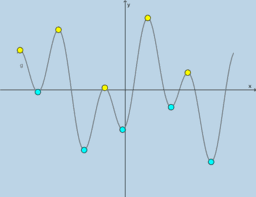Algebra 2 resources

Book

Bart Landenberger

•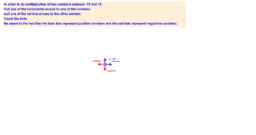Algebra 1

Book

Bart Landenberger

•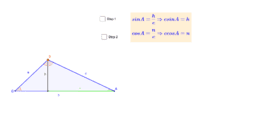Law of Cosines

Activity

Bart Landenberger

•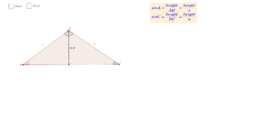Law of Sines

Activity

Bart Landenberger

•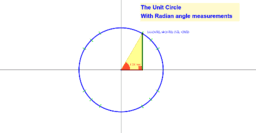Unit Circle with Randian Angle Measurements

Activity

Bart Landenberger

•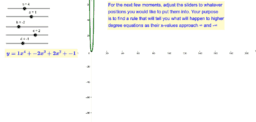End Behavior

Activity

Bart Landenberger

•_|_ through a point #1

Activity

Bart Landenberger

•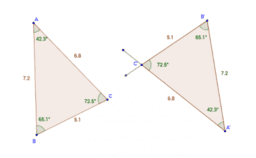Exploring Triangle Congruence

Book

Bart Landenberger

•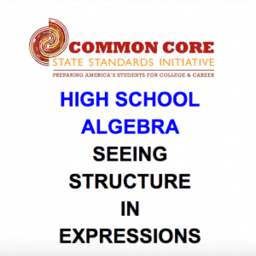CCSS High School: Algebra (Seeing Structure in Expressions)

Book

Bart Landenberger

•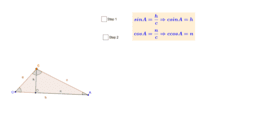Law of Cosines

Activity

Bart Landenberger

•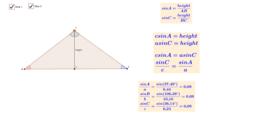Law of Sines

Activity

Bart Landenberger

•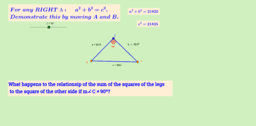Pythagorean Theorem and Converse

Activity

Bart Landenberger

•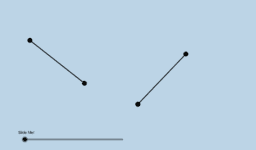CCSS High School: Geometry (Congruence) Volume 1

Book

Bart Landenberger

•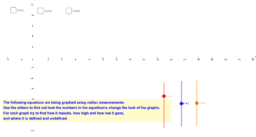sin, cos, tan graphs

Activity

Bart Landenberger

•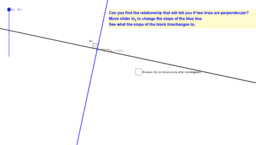equations of perpendicular lines

Activity

Bart Landenberger

•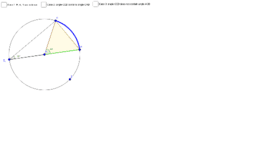Proof - inscribed angle

Activity

Bart Landenberger

•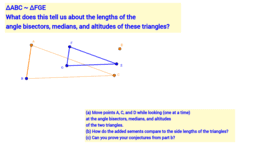Segments in similar triangles

Activity

Bart Landenberger

•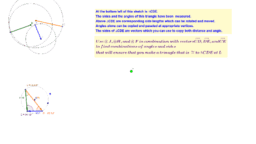Triangle Congruence Tester

Activity

Bart Landenberger

•Multiplying positive and negative numbers.

Activity

Bart Landenberger

•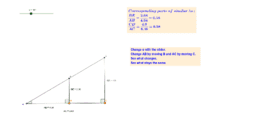Tangent Demonstration

Activity

Bart Landenberger

•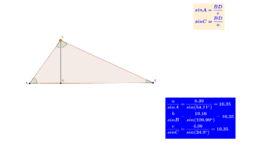Law of Sines

Activity

Bart Landenberger

•Angle Bisector Construction

Activity

Bart Landenberger

•Perpendicular Bisector Construction

Activity

Bart Landenberger

•Perpendicular through a point #2

Activity

Bart Landenberger